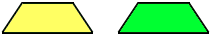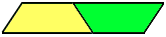Subject: isosceles trapezoid formula
Date: Tue, 12 May 1998 11:06:11 -0400

Dear Math Central,

The teacher of Gifted and Talented Math has been trying to locate the formula for anisosceles trapezoid and we can't find it anywhere. Could it be the same formula for that of a parallelogram ? Please advise.

Many thanks !

Donna McMullin
Media Specialist

Hi Donna,
 The AREA of an isosceles triangle is found by taking 1/2 the sum of the long and short parallel sides, times the height. One way to compare this with the parallelogram is to take two copies, turn one 180 degrees and glue them along the equal (nonparallel) edges. You now have a larger parallelogram - with one side composed of the sum of the original short and long parallel edges. Use the usual formula for the area of this parallelogram, then divide by 2 (since it is composed of two equal copies).Walter Whiteley
York University

Go to Math Central

To return to the previous page use your browser's back button.